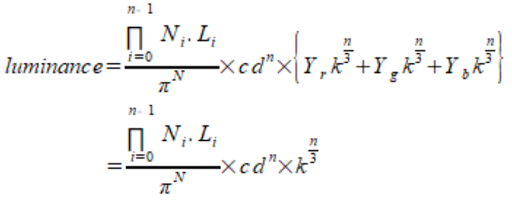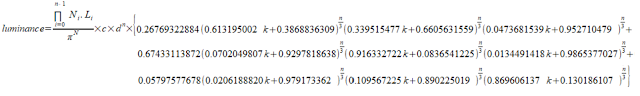### sRGB/ACEScg Luminance Comparison

Introduction

When I was searching information about rendering in different color spaces, I came across that using wider color primaries  (e.g. ACEScg instead of sRGB/Rec709) to perform lighting calculation will have a result closer to spectral rendering. But will this affect the brightness of the bounced light? So I decided to find it out. (The math is a bit lengthy, please feel free to skip to the result.)

Comparison method

To predict the brightness of the rendered image, we can consider the reflected light color after n bounces. To simplify the problem, we assume all the surfaces are diffuse material. We can derive a formula for the RGB color vector c after n light bounces.

To calculate lighting in different color spaces, we need to convert the albedo and initial light color to our desired color gamut by multiplying with a matrix M (For rendering in sRGB/Rec709, M is identity matrix).

Finally, we can calculate the luminance of the bounced light by computing the dot product between the color vector c and luminance vector Y of the color space (i.e. Y is the second row vector of the conversion matrix from a color space to XYZ space, with chromatic adaptation).

Now, we have an equation to compute the brightness of a reflected ray after n bounces in arbitrary color space.

Grey Material Test

To further simplify the problem, we assume all the surfaces are using the same material:

Then assuming all the surfaces are grey in color:

Now, the luminance equation is simpler to understand.

Substituting the matrix M and luminance vector Y for sRGB color gamut, the equation is very simple:

Then we do the same thing for ACEScg. Surprisingly, there are some constants roughly equal to one, so we can approximate them with constant one and the result will roughly equal to the luminance equation of sRGB.

As both equations are roughly equal, the rendered images in sRGB and ACEScg should be similar. Let's try to render images in sRGB and ACEScg to see the result (images are path traced with sRGB and ACEScg primaries, and then displayed in sRGB).

Both images looks very similar! So rendering in different color spaces with grey material will not change the brightness of the image. At least, the difference is very small after tone mapped to a displayable range.

Red Material Test

Now, let's try to use red material instead of grey material to see how the luminance changes (where k is a variable to control how 'red' the material is):

But the equation is still a bit complex,  so we further assume the initial light color is white in color.

Then we perform the same steps in last section, substituting M and Y into luminance equation.

Unfortunately, both equations are a bit too complex to compare, having 2 variables k and n... May be let's try to plot some graphs to see how those variables affect the luminance, with number of bounced light = 3 and 5 (i.e. n=3 and n=5, skipping the N dot L part because both equations have such term). From the graphs below: when k increases (i.e. the red color is getting more saturated, with RGB value closer to (1, 0, 0) ), luminance difference will increase, hence sRGB will have a larger luminance value than ACEScg.

Then comparing the images rendered in sRGB and ACEScg:

The indirectly lit area looks much brighter when rendered in sRGB. This makes sense because for any red color, its red channel value will be closer to one (while green/blue values will be closer to 0) when represented in sRGB compared to be represented in ACEScg. After several multiplication, the reflected light value should be larger when computation is done in sRGB.

RGB Material Test

How about using different colored material this time? Assuming 1/3 of the light bounced on surface that is red material, 1/3 is green material, and 1/3 is blue material.

Like previous 2 sections, substituting M and Y, the luminance equation becomes:sRGB luminance equation ACEScg luminance equation

And then plotting graphs to see how k and n varies:

The result is different this time. The sRGB luminance is smaller than ACEScg luminance, and the difference increases when both k and n increases. So the bounced light will be darker when rendered in sRGB.

Let's try rendering some images to see whether this is true. Although we cannot force the ray to bounce with 1/3 red/green/blue material exactly, I roughly assigned 1/3 of the material in red/green/blue color in the scene.

From the screen shots above, the indirectly lit red material looks darker when rendered in sRGB (especially the curtains on the ground floor), while the differences for the blue and green material are small. We can think of the result like previous section, for a given red color, when it is represented in sRGB, its red channel value is closer to one, however, its blue and green channels values are closer to 0 compared to be represented in ACEScg (same for both blue and green material). So after several multiplication with different color material, the RGB values in sRGB may becomes closer to 0 because different color material cancel out each other (e.g. when light bounced on red and green albedo surface (1, 0, 0) , (0, 1, 0) in sRGB, the reflected light will be zero, while the same color represented in ACEScg, the color will not be "zeroed out"), resulting in darker image.

Conclusion

After testing with different assumptions, the brightness of images when rendered in sRGB can be darker, roughly equal or brighter than rendered in ACEScg. The brightness difference depends on material used in the scene. If the scene uses grey material only, brightness will be equal. If material has similar color (e.g. all red material), sRGB image will be brighter. If the scene has more color variation, the sRGB image may becomes darker. And turns out this conclusion can be arrived without doing such lengthy math. We can think of the same color value represented in sRGB and ACEScg space: Is the RGB value closer to 0 or 1 when represented in the color space? Will the RGB values 'cancel' each other when performing lighting calculation in the color space? So I was too stupid to figure out this simple answer early and instead worked on such lengthy math... >.<

Reference